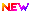# GATE 2020 Statistics Syllabus (Released) – Get (ST) PDF Here

Latest Applications Open 2020:GATE 2020 Syllabus of Statistics has been Released. GATE 2020 is a national level exam organized by IIT Delhi. The Engineering Graduation Skill Test (GATE 2020) is organized for admission to PG courses in the field of engineering and technology, specifically ME / M.Tech.

The Exam will be held on 1st, 2nd, 8th February and 9th February 2020. See here for complete information about the GATE 2020 Syllabus.

## GATE 2020 Statistics Syllabus – PDF ReleasedGATE 2020 Statistics Syllabus has been Released. Click Here to Download PDF.

## GATE 2020 Statistics Syllabus

Latest Applications For Various UG & PG Courses Open 2020:

1. Lovely Professional University| Admissions Open for All Courses 2020-21. Apply Now
2. Chandigarh University, Punjab | Admissions Open for All Courses 2020-21. Apply Now
3. Manipal University 2020 Admission Open For UG & PG Courses. Apply Now
4. UPES, Dehradun Enroll Yourself for the Academic Year 2020. Apply Now
5. Pearl Academy No.1 Fashion School – Admissions Open for 2020 batch. Apply Now

Calculus: Finite, countable and uncountable sets, Real number system as a complete ordered field, Archimedean property; Sequences and series, convergence; Limits, continuity, uniform continuity, differentiability, mean value theorems; Riemann integration, Improper integrals; Functions of two or three variables, continuity, directional derivatives, partial derivatives, total derivative,

maxima and minima, saddle point, a method of Lagrange’s multipliers; Double and Triple integrals and their applications; Line integrals and Surface
integrals, Green’s theorem, Stokes’ theorem, and Gauss divergence theorem.

Linear Algebra: Finite dimensional vector spaces over real or complex fields; Linear transformations and their matrix representations, rank; systems of linear equations, eigenvalues and eigenvectors, minimal polynomial, Cayley-Hamilton Theorem, diagonalization, Jordan canonical form,

symmetric, skew-symmetric, Hermitian, skew-Hermitian, orthogonal and unitary matrices; Finite dimensional inner product spaces, Gram- Schmidt orthonormalization process, definite forms.

Probability: Classical, relative frequency and axiomatic definitions of probability, conditional probability, Bayes’ theorem, independent events; Random variables and probability distributions, moments and moment generating functions, quantiles; Standard discrete and continuous univariate distributions;

Probability inequalities (Chebyshev, Markov, Jensen); Function of a random variable; Jointly distributed random variables, marginal and conditional distributions, product moments, joint moment generating
functions, independence of random variables; Transformations of random variables, sampling distributions, distribution of order statistics and range; Characteristic functions; Modes of convergence; Weak and strong laws of large numbers; Central limit theorem for i.i.d. random variables with the existence of higher-order moments.

Stochastic Processes: Markov chains with finite and countable state space, classification of states, limiting behavior of n-step transition probabilities, stationary distribution, Poisson and birth-and-death processes.

Inference: Unbiasedness, consistency, sufficiency, completeness, uniformly minimum variance unbiased estimation, method of moments and maximum likelihood estimations; Confidence intervals; Tests of hypotheses, most powerful and uniformly most powerful tests, likelihood ratio tests, large sample test, Sign test, Wilcoxon signed-rank test, Mann- Whitney U test, test for independence and Chi-square test for goodness of fit.

Regression Analysis: Simple and multiple linear regression, polynomial regression, estimation, confidence intervals and testing for regression coefficients; Partial and multiple correlation coefficients.

Multivariate Analysis: Basic properties of the multivariate normal distribution; Multinomial distribution; Wishart distribution; Hotelling’s T2 and related tests; Principal component analysis; Discriminant analysis; Clustering.

Design of Experiments: One and two-way ANOVA, CRD, RBD, LSD, 22 and 23 Factorial Experiments.

If you any query regarding GATE 2020 Statistics Syllabus, you can ask your query leave comments below.Lovely Professional University+